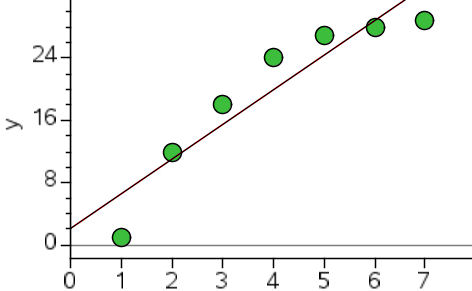2612 Scatter Plot Transformation Squared

15 Questions | Total Attempts: 300SettingsX and y squared transformations

• 1.
The y squared transformation linearises the scatter plot by?
• A.

Squaring the y axis values

• B.

Stretching the y axis value

• C.

Squaring the x axis values

• D.

Stretching the x axis value

• 2.
The x squared transformation linearises the scatter plot by?
• A.

Squaring the y axis values

• B.

Stretching the y axis value

• C.

Squaring the x axis values

• D.

Stretching the x axis value

• 3.
The scatter plot above will potentially be linearised with :
• A.

X squared transformation

• B.

Y squared transformation

• C.

It is is the wrong quadrant for these transformations

• 4.
The scatter plot above will potentially be linearised with :
• A.

X squared transformation

• B.

Y squared transformation

• C.

It is is the wrong quadrant for these transformations

• 5.
The scatter plot above will potentially be linearised with :
• A.

X squared transformation

• B.

Y squared transformation

• C.

It is is the wrong quadrant for these transformations

• 6.
The scatter plot above will potentially be linearised with :
• A.

X squared transformation

• B.

Y squared transformation

• C.

It is is the wrong quadrant for these transformations

• 7.
Which quadrants have potential for an x squared transformation
• A.

• B.

• C.

• D.

• 8.
Which quadrants have potential for a y squared transformation
• A.

• B.

• C.

• D.

• 9.
The residual plot above shows the original data is potentially from which quadrants
• A.

• B.

• C.

• D.

• 10.
The residual plot above shows the original data is potentially from which quadrants
• A.

• B.

• C.

• D.

• 11.
The residual plot above shows the original data can potentially be linearised with :
• A.

X squared transformation

• B.

Y squared transformation

• C.

It can't be linearised with a squared transformation

• 12.
The residual plot above shows the original data can potentially be linearised with :
• A.

X squared transformation

• B.

Y squared transformation

• C.

It can't be linearised with a squared transformation

• 13.
The residual plot above shows the original data can potentially be linearised with :
• A.

X squared transformation

• B.

Y squared transformation

• C.

It can't be linearised with a squared transformation

• 14.
The squared transformations are not applicable in which quadrant?
• A.

• B.

• C.

• D.

• E.

• 15.
The image above is a transformer from :
• A.

• B.

• C.Back to top